Courses

# Fill Ups, True/False, of Electromagnetic Induction and Alternating Current, Past year Questions JEE JEE Notes | EduRev

## Class 12 Physics 35 Years JEE Mains &Advance Past year Paper

Created by: Abhishek Kapoor

## JEE : Fill Ups, True/False, of Electromagnetic Induction and Alternating Current, Past year Questions JEE JEE Notes | EduRev

The document Fill Ups, True/False, of Electromagnetic Induction and Alternating Current, Past year Questions JEE JEE Notes | EduRev is a part of the JEE Course Class 12 Physics 35 Years JEE Mains &Advance Past year Paper.
All you need of JEE at this link: JEE

Fill in the Blanks

Q.1. A uniformly wound solen oidal coil of self inductance 1.8 × 10–4 henry and resistance 6 ohm is broken up into two identical coils. These identical coils are then connected in parallel across a 15-volt battery of negligible resistance. The time constant for the current in the circuit is ........... seconds and the steady state current through the battery is ............... amperes.       (1989 - 2 Marks)

Ans. 0.3 × 10–4 sec, 10 A

Solution. The coil is broken into two identical coils.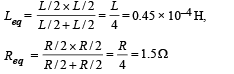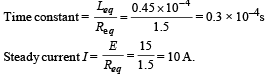Q.2. In a straight conducting wire, a constant current is flowing from left to right due to a source of emf. When the source is switched off, the direction of the induced current in the wire will ................     (1993 - 1 Marks)

Ans. Left to right

Solution. NOTE : As the source is switched off, the current decreases to zero. The induced current opposes the cause as per Lenz's law. Therefore, the induced current will direct from left to right.

True/ False

Q.1. An e.m.f. can be induced between the two ends of a straight copper wire when it is moved through a uniform magnetic field.        (1980)

Ans. T

Solution. True. A copper wire consists of billions and billions of free electrons. When the wire is at rest, the average velocity of each electron is zero. But when the wire is in motion, the electrons have a net velocity in the direction of motion.

NOTE : A charged particle moving in a magnetic field experiences a force given by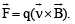Here also each electron experiences a force and therefore, electrons will move towards one end creating an emf between the two ends of a straight copper wire.

Q.2. A coil of metal wire is kept stationary in a non-uniform magnetic field. An e.m.f. is induced in the coil.     (1986 - 3 Marks)

Ans. F

Solution.NOTE : For induced emf to develop in a coil, the magnetic flux through it must change.

But in this case the number of magnetic lines of force through the coil is not changing. Therefore the statement is false.

Q.3. A conducting rod AB moves parallel to the x-axis (see Fig.) in a uniform magnetic field pointing in the positive z-direction. The end A of the rod gets positively charged.         (1987 - 2 Marks)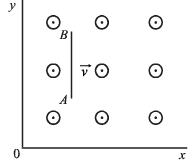Ans. T

Solution. NOTE : When conduction rod AB moves parallel to x-axis in a uniform magnetic field pointing in the positive z-direction, then according to Fleming’s left hand rule, the electrons will experience a force towards B. Hence, the end A will become positive.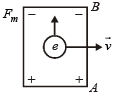Offer running on EduRev: Apply code STAYHOME200 to get INR 200 off on our premium plan EduRev Infinity!

42 docs|19 tests

,

,

,

,

,

,

,

,

,

,

,

,

,

,

,

,

,

,

,

,

,

,

,

,

,

,

,

,

,

,

;